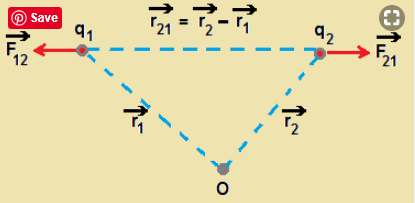# Derive coulombs law in vector form_______

Derive coulombs law in vector form_______.

Coulomb measured the force between two point charges and found that it varied inversly as the square of the distance between the charges and was directly proportional to the product of the magnitude of two charges and acted along the line of joining the two charges.
Thus if two point charges q1 and q2 are separated by a distance r in vacuum,
the magnitude of the force F between them is given by,…(1)

The constant k in eqn.(1) is usually put as, so that Coulombs law is written as…(2)

where εo is permitivity of free space and it is given by, εo = 8.854×10-12 C2N-1m-2
Since force is vector, we need to write Coulombs law in vector notation.vector quantity is given in Bold)

Let the position vectors of charges q1 and q2 be r1 and r2 respectively. We denote force on q1 due to q2 by F12 and

force on q2 by q1 by F21 as shown in figure. The two point charges q1 and q2 have been numbered 1 and 2 for conveneience

and the vector leading from 1 to 2 is denoted by r21

r21 = r2-r1 . The magnitude of vector r21 is denoted by | r21 |. The direction of a vector is specified by unit vector along that vector.

We define the unit vectorCoulombs force law between two point charges q1 and q2 located at r1 and r2 is then expressed asThe above equation is valid for any sign of q1 and q2. If q1 and q2 are of same sign, F21 is along r21 , which denotes repulsion.

If they are opposite sign, F21 is along -r21 that denotes attraction. No need to write separate equation for like and unlike charges